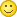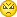# RGB Matrix module 20x40 1/5 scan GKGD - error of display of the image

This site uses cookies. By continuing to browse this site, you are agreeing to our Cookie Policy.

• Sure you dont use a vertical creating 'Set_text_12_16'?
Please try Call Set_text_6_8(0 , 0 , Green) in the same code
• ### Pluto25 wrote:

Sure you dont use a vertical creating 'Set_text_12_16'?
Of course!

### Pluto25 wrote:

Please try Call Set_text_6_8(0 , 0 , Green) in the same code
Vertical font or horizontal font?
• Const Dy_num_vertik = 1
Const Dy_num_horiz = 3
you mean?
•- black screen.

And new picture.

### Source Code

1. Reds_1:
2. Data &B11111111 , &B11111111 , &B11111111 , &B11111111 , &B11111111 , &B11111111 , &B11111111 , &B11111111 , &B11111111 , &B11111111 , &B11111111 , &B11111111 , &B11111111 , &B11111111 , &B11111111
3. Data &B10000000 , &B00000000 , &B00000000 , &B00000000 , &B00000000 , &B10000000 , &B00000000 , &B00000000 , &B00000000 , &B00000000 , &B00000000 , &B00000000 , &B00000000 , &B00000000 , &B00000001
4. Data &B10000000 , &B00000000 , &B00000000 , &B00000000 , &B00000001 , &B10000000 , &B00000000 , &B00000000 , &B00000000 , &B00000000 , &B00000000 , &B00000000 , &B00000000 , &B00000000 , &B00000001
5. Data &B10000000 , &B00000000 , &B00000000 , &B00000000 , &B00000001 , &B10000000 , &B00000000 , &B00000000 , &B00000000 , &B00000000 , &B00000000 , &B00000000 , &B00000000 , &B00000000 , &B00000001
6. Data &B10000000 , &B00000000 , &B00000000 , &B00000000 , &B00000001 , &B10000000 , &B00000000 , &B00000000 , &B00000000 , &B00000000 , &B00000000 , &B00000000 , &B00000000 , &B00000000 , &B00000001
7. Data &B10000000 , &B00000000 , &B00000000 , &B00000000 , &B00000001 , &B10000000 , &B00000000 , &B00000000 , &B00000000 , &B00000000 , &B00000000 , &B00000000 , &B00000000 , &B00000000 , &B00000001
8. Data &B10000000 , &B00000000 , &B00000000 , &B00000000 , &B00000001 , &B10000000 , &B00000000 , &B00000000 , &B00000000 , &B00000000 , &B00000000 , &B00000000 , &B00000000 , &B00000000 , &B00000001
9. Data &B10000000 , &B00000000 , &B00000000 , &B00000000 , &B00000001 , &B10000000 , &B00000000 , &B00000000 , &B00000000 , &B00000000 , &B00000000 , &B00000000 , &B00000000 , &B00000000 , &B00000001
10. Data &B10000000 , &B00000000 , &B00000000 , &B00000000 , &B00000001 , &B10000000 , &B00000000 , &B00000000 , &B00000000 , &B00000000 , &B00000000 , &B00000000 , &B00000000 , &B00000000 , &B00000001
11. Data &B10000000 , &B00000000 , &B00000000 , &B00000000 , &B00000001 , &B10000000 , &B00000000 , &B00000000 , &B00000000 , &B00000000 , &B00000000 , &B00000000 , &B00000000 , &B00000000 , &B00000001
12. Data &B10000000 , &B00000000 , &B00000000 , &B00000000 , &B00000001 , &B10000000 , &B00000000 , &B00000000 , &B00000000 , &B00000000 , &B00000000 , &B00000000 , &B00000000 , &B00000000 , &B00000001
13. Data &B10000000 , &B00000000 , &B00000000 , &B00000000 , &B00000001 , &B10000000 , &B00000000 , &B00000000 , &B00000000 , &B00000000 , &B00000000 , &B00000000 , &B00000000 , &B00000000 , &B00000001
14. Data &B10000000 , &B00000000 , &B00000000 , &B00000000 , &B00000001 , &B10000000 , &B00000000 , &B00000000 , &B00000000 , &B00000000 , &B00000000 , &B00000000 , &B00000000 , &B00000000 , &B00000001
15. Data &B10000000 , &B00000000 , &B00000000 , &B00000000 , &B00000001 , &B10000000 , &B00000000 , &B00000000 , &B00000000 , &B00000000 , &B00000000 , &B00000000 , &B00000000 , &B00000000 , &B00000001
16. Data &B10000000 , &B00000000 , &B00000000 , &B00000000 , &B00000001 , &B10000000 , &B00000000 , &B00000000 , &B00000000 , &B00000000 , &B00000000 , &B00000000 , &B00000000 , &B00000000 , &B00000001
17. Data &B10000000 , &B00000000 , &B00000000 , &B00000000 , &B00000001 , &B10000000 , &B00000000 , &B00000000 , &B00000000 , &B00000000 , &B00000000 , &B00000000 , &B00000000 , &B00000000 , &B00000001
18. Data &B10000000 , &B00000000 , &B00000000 , &B00000000 , &B00000001 , &B10000000 , &B00000000 , &B00000000 , &B00000000 , &B00000000 , &B00000000 , &B00000000 , &B00000000 , &B00000000 , &B00000001
19. Data &B10000000 , &B00000000 , &B00000000 , &B00000000 , &B00000001 , &B10000000 , &B00000000 , &B00000000 , &B00000000 , &B00000000 , &B00000000 , &B00000000 , &B00000000 , &B00000000 , &B00000001
20. Data &B10000000 , &B00000000 , &B00000000 , &B00000000 , &B00000001 , &B10000000 , &B00000000 , &B00000000 , &B00000000 , &B00000000 , &B00000000 , &B00000000 , &B00000000 , &B00000000 , &B00000001
21. Data &B11111111 , &B11111111 , &B11111111 , &B11111111 , &B11111111 , &B11111111 , &B11111111 , &B11111111 , &B11111111 , &B11111111 , &B11111111 , &B11111111 , &B11111111 , &B11111111 , &B11111111
Display All
True picture:
3-GKGD_007-true.jpg
Real:
3-GKGD_008.jpg
• Perhaps the code for horizontal connection of matrices and vertical connection will be different?
Can I use this code?
But so far it does not work more than 1 matrix

Although for Qjang Li matrices, I use the same code. Changing only the number of vertical and horizontal matrices.
Files

The post was edited 1 time, last by SAMEGO ().

• reds_1 shold work with
Const Dy_num_vertik = 3
Const Dy_num_horiz = 1
num_matr can't do his job so the code is now workingThe post was edited 1 time, last by Pluto25 ().

• the code from 227 can't work more the one Panel. Try the one from #216 with wrong settings (#228)
• I think I need to redo the code in which the display starts based on the number of matrices (as in the code for QL). Tomorrow I will try.

### Source Code

1. If Page = 0 Then
2. Mem_offset_1 = 0 : Mem_offset_3 = 5 * Bytes_in_row 'page=1
3. End If
4. If Page = 1 Then
5. Mem_offset_1 = Bytes_in_row : Mem_offset_3 = 6 * Bytes_in_row 'page=2
6. End If
7. If Page = 2 Then
8. Mem_offset_1 = 2 * Bytes_in_row : Mem_offset_3 = 7 * Bytes_in_row 'page=3
9. End If
10. If Page = 3 Then
11. Mem_offset_1 = 3 * Bytes_in_row : Mem_offset_3 = 8 * Bytes_in_row 'page=4
12. End If
13. If Page = 4 Then
14. Mem_offset_1 = 4 * Bytes_in_row : Mem_offset_3 = 9 * Bytes_in_row 'page=5
15. End If
16. For R_0 = 1 To Dy_num_h
17. St_byte_1 = Mem_offset_3 : St_byte_2 = St_byte_1 + Channel_offset 'set start-bytes
18. St_byte_3 = Mem_offset_1 : St_byte_4 = St_byte_3 + Channel_offset
19. For B_1 = 1 To Bytes_in_row
Display All
• ### Pluto25 wrote:

the code from 227 can't work more the one Panel. Try the one from #216 with wrong settings (#228)

### Source Code

1. Const Dy_num_vertik = 3
2. Const Dy_num_horiz = 1

### Source Code

1. Text = "0123456789"
2. Call Set_text_12_16(0 , 0 , Red)

3-GKGD_007.jpg

### Source Code

1. Text = "0123456789"
2. Call Set_text_12_16(0 , 21 , Red)
3-GKGD_007_2.jpg

### Source Code

1. Text = "0123456789"
2. Call Set_text_12_16(0 , 41 , Red)
3-GKGD_007_3.jpg

The post was edited 2 times, last by SAMEGO ().

• Please show reds_1 and some pictures they normaly works on horizontale Panels .
And some Text Call Set_text_6_8(0 , 0 , Green) - horizont SUB
bevore we change anything in code we must know if its only a error in Set_text_12_16
The panel conected to atmega is left? (last images) And it was left booton '3-GKGD_005.jpg' (#216)?
• ### Pluto25 wrote:

Please show reds_1 and some pictures they normaly works on horizontale Panels .
I will make in the evening

### Pluto25 wrote:

And some Text Call Set_text_6_8(0 , 0 , Green) - horizont SUB
3-GKGD_009.jpg

### Source Code

1. '20x40 Ledmatrixes RGB
2. '40 leds=5 bytes, left-led of a byte=byte.7, right-led of a byte=byte.0
3. ' ---------------- ---------------- ----------------
4. ' | | | | | |
5. ' | | | | | |
6. ' | |<<<| |<<<| |<<<
7. ' | | | | | | ^
8. ' | | | | | | ^
9. ' ---------------- ---------------- ---------------- ^
10. ' ^
11. ' >>>>>>>>>>>>>>>>>>>>>>>>>>>>>>>>>>>>>>>>>>>>>>>>>>>>>>>>>>
12. ' ^
13. ' ^ ---------------- ---------------- ----------------
14. ' ^ | | | | | |
15. ' ^ | | | | | |
16. ' ^ <| |<<<| |<<<| |<<< data in
17. ' | | | | | |
18. ' | | | | | |
19. ' X--------------- ---------------- ----------------
20. ' x=0, y=0 in the left down corner
Display All

### Pluto25 wrote:

The panel conected to atmega is left? (last images)
When viewed from the front, the controller is on the right.
Yes.

The post was edited 1 time, last by SAMEGO ().

• Try this with correct
Const Dy_num_vertik = 1
Const Dy_num_horiz = 3

### Source Code

1. Dim c_0 as Byte
2. Display_refresh:
4. If Page_counter < 4 Then
5. Incr Page_counter
6. Else
7. Page_counter = 0
8. End If
9. For R_0 = 1 To Dy_num_vertik
10. Atemp = R_0 - 1
11. Atemp = Atemp * 100
12. Index_1 = Page_counter * 5
13. Index_1 = Index_1 + Atemp
14. For C_0 = Dy_num_horz To 1
15. Atemp = C_0 - 1
16. Atemp = Atemp * 100
17. Atemp = 0
18. For D_1 = 1 To 10
19. Index_2 = Index_1 + 50
20. If D_1.0 = 1 Then
21. Shift6out Memory_red(index_1) , Memory_green(index_1) , Memory_blue(index_1) , Memory_red(index_2) , Memory_green(index_2) , Memory_blue(index_2)
22. Else
23. Shift6lout Memory_red(index_1) , Memory_green(index_1) , Memory_blue(index_1) , Memory_red(index_2) , Memory_green(index_2) , Memory_blue(index_2)
24. End If
25. If Atemp = 0 Then
26. Index_1 = Index_1 + 25
27. Incr Atemp
28. Else
29. Index_1 = Index_1 - 24
30. Atemp = 0
31. End If
32. Next D_1
33. Next C_0
34. Next R_0
35. 'Trigger = 0
36. Pwm1b = 0
37. Waitus 100
38. P_lat = 1 : P_lat = 0
39. P_a = Page_counter.0
40. P_b = Page_counter.1
41. P_c = Page_counter.2
42. Pwm1b = Brightness
43. Trigger = 1
44. Return
Display All
• code from 227 and up correct and
Gosub Clear_memory
Text = "437"
Call Set_text_12_16(0 , 0 , Red)
Wait 1
Gosub Clear_memory
Text = "Test Bascom AVR "
Call Set_text_6_8(0 , 0 , Green)
Wait 1

Black screen. There is no image.
• This shoud't make black

### Source Code

1. Display_refresh:
3. If Page_counter < 4 Then
4. Incr Page_counter
5. Else
6. Page_counter = 0
7. End If
8. For R_0 = 1 To Dy_num_vertik
9. Index_1 = Page_counter * 5
10. Atemp = R_0 - 1
11. Atemp = Atemp * 100
12. Index_1 = Index_1 + Atemp
13. For C_0 = Dy_num_horiz To 1 Step -1
14. Atemp = C_0 - 1
15. Atemp = Atemp * 100
16. Index_1 = Index_1 + Atemp
17. Atemp = 0
18. For D_1 = 1 To 10
19. Index_2 = Index_1 + 50
20. If D_1.0 = 1 Then
21. Shift6out Memory_red(index_1) , Memory_green(index_1) , Memory_blue(index_1) , Memory_red(index_2) , Memory_green(index_2) , Memory_blue(index_2)
22. Else
23. Shift6lout Memory_red(index_1) , Memory_green(index_1) , Memory_blue(index_1) , Memory_red(index_2) , Memory_green(index_2) , Memory_blue(index_2)
24. End If
25. If Atemp = 0 Then
26. Index_1 = Index_1 + 25
27. Incr Atemp
28. Else
29. Index_1 = Index_1 - 24
30. Atemp = 0
31. End If
32. Next D_1
33. Next C_0
34. Next R_0
35. 'Trigger = 0
36. Pwm1b = 0
37. Waitus 100
38. P_lat = 1 : P_lat = 0
39. P_a = Page_counter.0
40. P_b = Page_counter.1
41. P_c = Page_counter.2
42. Pwm1b = Brightness
43. Trigger = 1
44. Return
Display All